# Respiratory System Mechanics and Respiratory Muscle Function

Filed under Critical Care Medicine

rate 1 star rate 2 star rate 3 star rate 4 star rate 5 star46 Respiratory System Mechanics and Respiratory Muscle Function

In its simplest form, the respiratory system can be modeled as a balloon connected to a tube. The balloon represents the elastic element (lungs and chest wall), and the tube represents the resistive element (conducting airways). To serve the purpose of ventilation, the respiratory pump (or a mechanical ventilator) must generate sufficient pressure to overcome both the elastic and flow-resistive properties of the respiratory system.

Classic respiratory mechanics are based on Newtonian physics, as expressed in the equation of motion. The respiratory system model is derived from an elementary monodimensional system, as depicted by a block with an attached spring, acted on by a unidirectional force (Figure 46-1, A).1,2 Upon application of force, the response of the system can be characterized in terms of displacement, velocity, and acceleration of a block with a mass of M. The balance of forces acting on the block can be expressed as follows: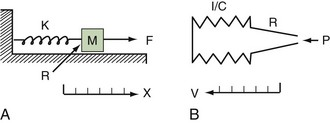(From Rodarte JR, Rehder K. Dynamics of respiration. In: Macklem PT, Mead J, editors. Handbook of Physiology. Baltimore: Williams & Wilkins; 1986:131-144.)

(Equation 1)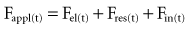where the total force applied to the system (Fappl) at a given time (t) is equal to the sum of the elastic (Fel), resistive (Fres), and inertial (Fin) forces.

The equation of motion for a three-dimensional pneumatic system may be written as:

(Equation 2)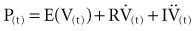where P(t) is the pressure exerted on the system at a given time; E is the elastance (the reciprocal of compliance, i.e., 1/C), which relates pressure to volume (V), and R is the resistance constant, relating pressure to flow. The third term of the equation describes the pressure required to accelerate tissue and gas in the airway, which is an important factor under certain circumstances such as coughing or high-frequency oscillatory ventilation. The inertance constant (I) relates pressure to linear acceleration (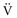). However, the third term is usually omitted in this model of the respiratory system, because inertive forces are negligible during quiet breathing and most forms of mechanical ventilation.3 Thus, in most applications, the respiratory system derivative of the equation of motion considers only the elastic and flow-resistive elements that oppose an applied pressure at time (t):

(Equation 3)which may also be expressed as:

(Equation 4)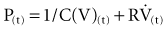In this model, any force applied to the respiratory system is either stored as elastic energy or dissipated as resistive energy. Figure 46-1, B shows a three-dimensional model of the respiratory system as it relates to the equation of motion.

This simple model of respiratory system mechanics is useful because, in the normal operating range, the relationships among airway pressure, volume, and flow can be approximated by straight lines. Linear one-compartment analogs are particularly well suited for modeling mechanical ventilation because the pressure applied to lungs and chest wall can be readily measured and displayed. In turn, departures from linearity provide useful clues about concurrent respiratory muscle activity, alert the healthcare provider to the presence of lung disease, or serve as a warning that the lungs are being ventilated at inappropriately high or low volumes.

#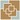Static Behavior of the Respiratory System

Static behavior of the respiratory system defines a condition in the absence of flow. Under such conditions, and in accordance with the model described in the preceding section, a pressure applied to the respiratory system is opposed by elastic forces (Pel). During flow, this pressure can be approximated by alveolar pressure (Palv) which, upon interruption of airflow, equilibrates with airway opening pressure (Pao):

(Equation 5)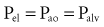The elastic element of the respiratory system (rs) consists of two component structures, the chest wall (w)—functionally, the thoracic cage and abdomen—and the lungs (l). The forces that act on these two structures can be summed, because the lungs and chest wall behave like springs in series:

(Equation 6)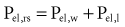The net distending pressure applied to the lung by contraction of the inspiratory muscles or by positive-pressure ventilation is represented by transmural forces, termed the transpulmonary pressure (PL), is determined by the difference between alveolar pressure (Palv) and pleural pressure (Ppl):

(Equation 7)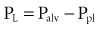The pressure across the chest wall (transthoracic pressure, Pw) is determined by the difference between pleural pressure and atmospheric pressure (Ppbs):

(Equation 8)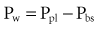Because it is used as a reference to all other measured pressures, atmospheric pressure is considered to be zero, thus:

(Equation 9)An esophageal balloon catheter can be used to approximate pleural pressure, keeping in mind that pleural pressure is nonuniform and that topographic gradients in pleural pressure vary with posture.4 In the recumbent posture, there is no site in the esophagus at which local pressure approximates average lung surface pressure (i.e., average pleural pressure). However, at least in normal lungs, the average change in surface or pleural pressure can be inferred using esophageal manometry.

The static pressure across the entire respiratory system in the absence of flow and assuming the pressure at the airway opening (Pao) equals alveolar pressure can be summarized as follows:

(Equation 10)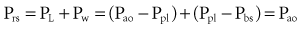By assuming that Pbs is considered to be zero:

(Equation 11)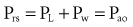The static respiratory system pressure-volume (P-V) curve is often measured in intubated, mechanically ventilated patients to make inferences about the mechanical properties of the lungs. Although the utility of P-V measurements in clinical decision making remains to be established, the determinants of the P-V relationship should nevertheless be understood. The P-V curve is generated by inflating and deflating the relaxed respiratory system in a stepwise fashion between residual volume and total lung capacity. The airway occlusion pressure at each volume defines the corresponding elastic recoil pressures of the lungs and chest wall. Because the inflation and deflation relationships differ from each other, the resulting curve is often referred to as a P-V loop. The respiratory system P-V loop is the summation of individual lung and chest wall P-V loops, termed a Rahn diagram (Figure 46-2). Because during normal tidal volume breathing (30% to 70% vital capacity), the relationship between elastic pressure and volume is essentially linear, the system’s elastic properties can be defined by a constant, namely elastance. The term compliance is more frequently used and is simply the inverse of elastance, defined as the change in volume per unit change in applied pressure. Static respiratory system compliance can be determined by the slope of the P-V curve. In the quiet breathing range, the normal respiratory system elastance averages 8 to10 cm H2O/L, corresponding to a static respiratory system compliance of 0.12 to 0.1 L/cm H2O.(From Agostoni E, Hyatt RE. Static behavior of the respiratory system. In: Fishman AP, editor. Handbook of Physiology. Baltimore: Williams & Wilkins; 1986:113-130.)

Figure 46-2 shows the P-V curves of the respiratory system’s component structures, the lung and chest wall. At high lung volumes, the total respiratory system compliance is reduced (the P-V curve is concave to the pressure axis), primarily because the lung reaches total capacity, its structural limit. In contrast, the P-V curve of the chest wall remains linear at high volumes (i.e., the chest wall offers much less resistance to further lung expansion).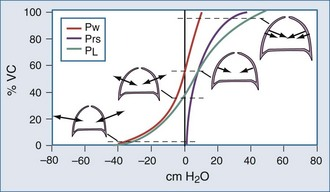(From Agostoni E, Hyatt RE. Static behavior of the respiratory system. In: Fishman AP, editor. Handbook of Physiology. Baltimore: Williams & Wilkins; 1986:113-130.)

Lung recoil is the collapsing force of the lung; it is in equilibrium with the transpulmonary distending pressure originating from the chest wall and inspiratory muscles and generated by:

As demonstrated in Figure 46-4, the elimination of surface tension has two important consequences on lung mechanics7: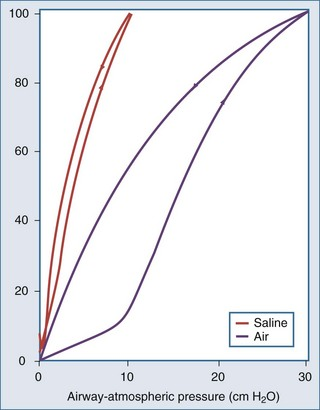(From Taylor A, Rehder K, Hyatt R, et al. Mechanics of breathing: static. In: Taylor AE, editor. Clinical Respiratory Physiology. Philadelphia: Saunders; 1989:89-105.)

Findings indicate not only that surface tension is an important source of lung elastic recoil but also that recoil pressure varies with volume, volume history, and time.

In the normal lung, hysteresis is caused by volume- and time-dependent changes in the molecular composition and hence the biophysical properties of surfactant. Surfactant is a protein-enriched lipid film that coats air-liquid interfaces in distal lung units and lowers surface tension. Hysteresis implies that energy added to the system during inflation is not fully recovered during deflation. The hysteretic loss of energy does not scale with frequency and flow the way a Newtonian viscous resistance does, underscoring one of the many limitations of linear resistance-compliance circuits in modeling lung mechanics.8 Whereas interfacial phenomena are the primary source of hysteresis in the normal lung, alveolar recruitment and derecruitment are important sources of hysteresis in disease.

According to the law of Laplace, the pressure (P) required to inflate a bubble is directly related to the surface tension (T) and is inversely proportional to the radius of curvature (r):

(Equation 12)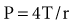Applied to the lung, this means that changes in alveolar dimensions at low lung volumes would promote alveolar collapse were it not for surfactant’s surface tension–lowering properties. A surfactant-depleted lung exhibits alveolar instability and collapse in the tidal breathing range. In normal pigs, high-tidal-volume ventilation does not alter alveolar mechanics in the normal lung; however, in the surfactant-deactivated lung, it causes alveolar overdistension and exacerbates alveolar instability.9 Figure 46-5 shows a pressure-volume loop of a normal lung and a surfactant-depleted lung. As a consequence of surfactant depletion, larger than normal transpulmonary pressures are required to keep the surfactant-depleted lung inflated.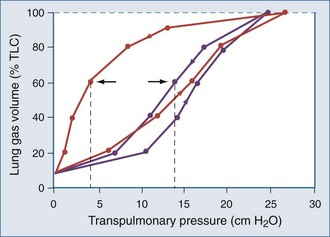(From Taylor A, Rehder K, Hyatt R, et al. Mechanics of breathing: static. In: Taylor AE, editor. Clinical Respiratory Physiology. Philadelphia: Saunders; 1989:89-105.)

#Dynamic Behavior of the Respiratory System

The transpulmonary pressure generated by the respiratory pump must overcome the resistive forces related to gas flow in order to generate a given volume in a given time. The respiratory system resistance constant scales resistive pressure and flow in the equation of motion discussed previously. The reciprocal of resistance is conductance, which is proportional to lung volume as the airways, tethered to the entire connective tissue network, are pulled open with larger inflation volumes.

According to Ohm’s law, resistance (R) can be calculated by dividing the driving pressure by flow:

(Equation 13)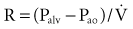Total pulmonary resistance reflects the gas flow–dependent pressure dissipation in the conducting airways (airway resistance) and the frequency-dependent loss of energy associated with parenchymal deformation (tissue resistance). Originally considered only a minor component of total pulmonary resistance, it is now appreciated that the so-called tissue resistance dominates the measurement, at least at low frequencies.10 As outlined by Fredberg and Stamenovic,8 tissue resistance and tissue hysteretic properties are model-specific descriptors of energy loss, the structural and molecular basis of which remains uncertain.11

The physical laws governing fluid flow in tubes can be applied to gas flow in the airways. According to fluid mechanics, tube length and geometry and gas velocity and physical properties (i.e., density and viscosity) determine whether flow is laminar or turbulent.

These determinants can be captured by Reynold’s number, a quantity that represents the ratio of inertial forces to viscous forces.12 A low Reynold’s number (<50) corresponds to laminar flow, and a Reynold’s number greater than 2300 is associated with turbulent flow. Accordingly, the low gas velocity in peripheral airways favors laminar flow, and the acceleration associated with the decrease in total cross-sectional area in central airways promotes turbulence.

In the presence of laminar flow, frictional pressure losses are linearly related to flow and viscosity and inversely proportional to tube radius to the fourth power (Poiseuille’s equation):

(Equation 14)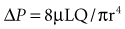where ΔP is the pressure drop, L is the length of pipe, µ is the dynamic viscosity, Q is the volumetric flow rate, r is the radius, and π is the mathematical constant (approximately 3.141592654). In contrast, turbulent flow is associated with nonlinear pressure-flow relationships that are gas-density dependent. The density dependence of turbulent flow is occasionally exploited in the medical use of heliox, a low-density helium-oxygen mixture given to patients with central airway lesions or asthma.13 However, the available clinical data on inhaled He/O2 mixtures are insufficient to prove that this therapy has benefit with respect to outcome variables.14

The flow-dependent shift from laminar to turbulent flow is captured in the Rohrer equation:

(Equation 15)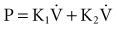where K1 and K2 are constants that scale frictional pressure dissipation associated with laminar and turbulent flow, respectively.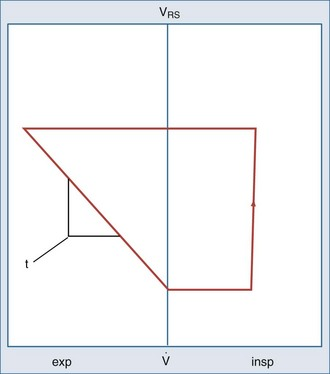(From Loring SH. Mechanics of the lung and chest wall. In: Marini JJ, Slutsky AS, editors. Physiological Basis of Ventilatory Support. New York: Marcel Dekker; 1998:177-205.)

(Equation 16)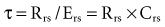where τ is usually measured in seconds because respiratory system resistance (Rrs) is expressed in units of pressure × time × volume−1, and respiratory system compliance (Crs) is expressed in units of volume × pressure−1.

The value of τ for a normal respiratory system is approximately 0.3 second.3 As can be inferred from the equation, patients with high respiratory system resistance or compliance, such as those with chronic obstructive lung disease (COPD), have correspondingly large τ values.

The added resistance of the artificial tubing in a mechanically ventilated patient may increase τ to 1 second or more. In addition, in patients with even minimally elevated τ values, the expiratory time (depending on the preset inspiratory time in time cycled ventilatory support or from the expiratory threshold in a flow cycled ventilatory mode15) may not be enough to fully empty the lungs during mechanical ventilation. Consequently, the demand for expiratory flow is not met as the lungs near relaxation volume, resulting in dynamic hyperinflation even in healthy lungs at high rates of respiratory frequency.

Another important concept that explains the dynamic behavior of the respiratory system is shown in Figure 46-7. Higher lung volumes (curve A) yield higher expiratory flow rates compared with the flow seen at lower lung volumes (curve C). In a classic set of experiments performed on normal subjects, Fry and Hyatt demonstrated that maximal expiratory flow is determined by lung volume.16 They concluded that on the basis of volume-related dynamic airway collapse, expiratory flow plateau cannot be exceeded irrespective of the magnitude of subject effort or applied transpulmonary pressure. Herein lies the value of the forced vital capacity maneuver as a reproducible measure of maximal expiratory flow.

#Assessment of Respiratory System Mechanics in the Intensive Care Unit

With the foundation described previously, we can proceed to the correlation of mechanics with clinical conditions encountered in the intensive care unit (ICU). To examine basic concepts, we use the example of expected waveforms generated by a volume-preset mechanical ventilator in a relaxed or paralyzed patient with otherwise normal respiratory system mechanics.

Assuming constant flow in a relaxed or paralyzed patient without respiratory muscle contribution, pressure at the ventilator inlet increases linearly with time and volume to a peak. A typical simplified waveform output is demonstrated by Figure 46-8, with a model of the system represented on the right. Pressures are measured at the ventilator inlet or at the airway opening at the level of the “Y” connection. Assuming inflation onset with a constant (square wave) flow, an initial step change in driving pressure is recorded, which precedes alveolar filling and corresponds to resistive pressure related to gas flow in the airways (see Figure 46-8, dotted arrow). When making an end-inspiration airway occlusion after a rapid initial step-off in resistive pressure drop (Pmax–P1), there is then a gradual decrease in pressure to a plateau value (P2) (see Figure 46-8, full thick arrows). This pressure, usually reached after 3 seconds of end-inspiratory occlusion, indicates the true static end-inspiratory elastic recoil pressure of the total respiratory system (Pst,rs)1718 and represents the static summation of elastic recoil forces corresponding to the applied tidal volume. During this period, the contribution in reduction in pressure due to volume loss by continuing gas exchange should be negligible.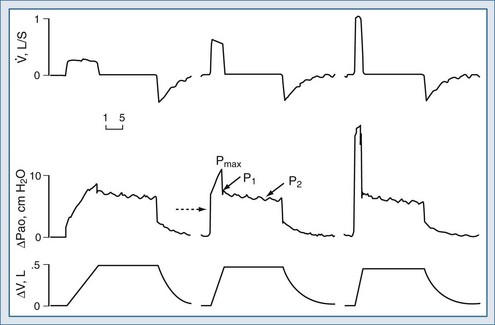(Modified from D’Angelo E, Calderini E, Torri G, et al. Respiratory mechanics in anesthetized paralyzed humans: effects of flow, volume, and time. J Appl Physiol. 1989;67:2556-64.)

The initial drop in Pmax, namely P1 divided by the preceding steady flow, provides the so-called ohmic resistances (Rmin,rs). Rmin,rs increases linearly with flow according to the Rohrer equation. The slow decrease of pressure (P1 − P2) divided by the preceding steady flow yields the effective additional resistance (ΔRs) due to the viscoelastic properties of the thoracic tissues and time constant inequalities within the lung and the chest wall (so-called pendelluft).19 The sum of Rmin,rs and ΔRs is defined as Rmax.rs.20 In a mechanically ventilated patient, where endotracheal tube resistance dominates measured total respiratory system resistance, the derived value of respiratory resistance must be interpreted with caution.

Artificial tubing is not a truly ohmic resistor, and estimates of resistance are highly dependent on inspiratory flow rates. Even after correction for tube size, high inspiratory resistance may be confounded by inspissated secretions or “tube biting.”

When using inspiratory flow settings of less than 1 L/sec with an endotracheal tube larger than 7 mm in internal diameter, resistive pressure (Rmin,rs) is usually less than 10 cm H2O. When peak airway pressure deviates from the inspiratory occlusion pressure (P1) by more than 10 cm H2O in a healthy subject, an increase in airway resistance should be suspected. In the absence of obvious intrinsic airway disease (acute or chronic), a ventilator hardware problem, tube kinking, or sputum retention should be suspected. However, more than the absolute value of the drop of pressure from peak to P1 deviation from the difference (Pmax − P1) in baseline value from the institution of mechanical ventilation may identify an airway resistive problem. It should also be kept in mind that a normal inspiratory resistance does not preclude the presence of expiratory resistance in severe airflow obstruction, as seen in COPD patients.

As previously mentioned, the elastic properties of the respiratory system can be determined from the slope of the P-V curve. Provided there is no contribution from respiratory muscles (as shown by a perfectly linear inspiratory P-V curve), the elastance (Ers), or reciprocal of compliance, can be derived from time-based curves with the following equation:

(Equation 17)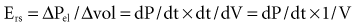where dP = change in pressure; dt = change in time; and dV = change in volume.

Elastance (Ers) can be measured at bedside during constant flow volume–preset mechanical ventilation. Pst,rs (P2 divided by ΔV) provides the static elastance of the total respiratory system (Est,rs):

(Equation 18)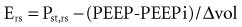where PEEP is the set end-expiratory positive pressure and PEEPi the intrinsic PEEP (see below).

As mentioned previously, during mechanical ventilation, the pressure applied to the respiratory system (Prs) overcomes PEEPi, flow resistance, and elastance of the respiratory system (Ers). Assuming a linear pressure volume curve, the total Ers (=1/compliance) equals the sum of the elastance of the chest wall (Ecw) and the lung (EL), which are mechanically in series:

(Equation 19)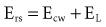Neglecting intrinsic PEEP and flow resistance, Prs is the sum of the pressures required to distend the chest wall (Pcw) and to inflate the lung (PL). The fractions EL/Ers and Ecw/Ers determine how Prs is partitioned between the lung:

(Equation 20)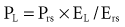and the chest wall:

(Equation 21)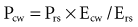For example, if the elastance of the chest wall is twice that of the lung, then two-thirds of Prs is used to distend the chest wall and only one-third to inflate the lung.21 Of note, the chest wall in this context comprises not only the thoracic rib cage but also the abdomen.

Compliance of the respiratory system can be also measured by the super syringe method. This method for static P-V curve recordings has been associated with spurious changes in lung volume because of gas absorption during measurement.22 There are also issues related to user interpretation (e.g., difficulties in defining morphologic characteristics of the curve), and interobserver variability is often high.23,24 Some have advocated inductive machine learning in an attempt to standardize interpretation.25

Passive expiration of the respiratory system is driven by elastic recoil, as manifested by alveolar pressure at a corresponding lung volume. Expiratory flow is a function of the elastance and resistance and is derived from the following relationship: